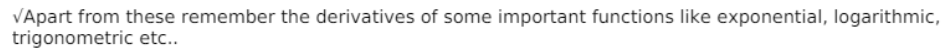# Find the second order derivatives of each of the following functions:

Question:

Find the second order derivatives of each of the following functions:

$\tan ^{-1} x$

Solution:

Basic idea:

$\sqrt{S e c o n d}$ order derivative is nothing but derivative of derivative i.e. $\frac{\mathrm{d}^{2} \mathrm{y}}{\mathrm{dx}^{2}}=\frac{\mathrm{d}}{\mathrm{dx}}\left(\frac{\mathrm{dy}}{\mathrm{dx}}\right)$

$\sqrt{T h e}$ idea of chain rule of differentiation: If $\mathrm{f}$ is any real-valued function which is the composition of two functions $u$ and $v$, i.e. $f=v(u(x))$. For the sake of simplicity just assume $t=u(x)$

Then $f=v(t)$. By chain rule, we can write the derivative of $f$ w.r.t to $x$ as:

$\frac{\mathrm{df}}{\mathrm{dx}}=\frac{\mathrm{dv}}{\mathrm{dt}} \times \frac{\mathrm{dt}}{\mathrm{dx}}$

$\sqrt{P r o d u c t}$ rule of differentiation- $\frac{d}{d x}(u v)=u \frac{d v}{d x}+v \frac{d u}{d x}$Let's solve now:

Given, $y=\tan ^{-1} x$

We have to find $\frac{d^{2} y}{d x^{2}}$